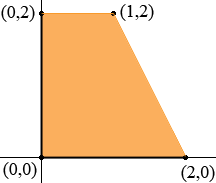SEARCH HOMEMath Central Quandaries & QueriesQuestion from alie, a student: a quadrilateral with exactly 1 pair of parallel sides and no congruent sides is what?Alie,

There is no specific word for quite this concept!

A quadrilateral with one pair of parallel sides is a trapezoid.
If it has two pairs of parallel sides it is a parallelogram, but parallelograms are also trapezoids in the same way that dogs are also mammals.

A parallelogram has two pairs of congruent sides. (They may all be congruent, in which case it is a rhombus, but a rhombus is also a parallelogram in the same way that a poodle is also a dog). Therefore the shape you describe is not a parallelogram.

If the two nonparallel sides of a nonparallelogram trapezoid are congruent, it is called an isosceles trapezoid, otherwise "scalene". The shape you describe is a scalene trapezoid; this may be what you want.

However, technically it is still not quite there, because this terminology does not deal with the question of whether one of the nonparallel sides is congruent to one of the parallel sides. For instance, the trapezoid with vertices (0,0),(2,0),(1,2) and (0,2) is scalene but has congruent sides.Note: Some sources define a trapezoid not to be a parallelogram, a parallelogram not to be a rhombus or a rectangle, and a rhombus or rectangle not to be a square, but this is a very bad idea; definitions like these are hard to work with. Be suspicious of any book that does this.

-RDMath Central is supported by the University of Regina and The Pacific Institute for the Mathematical Sciences.